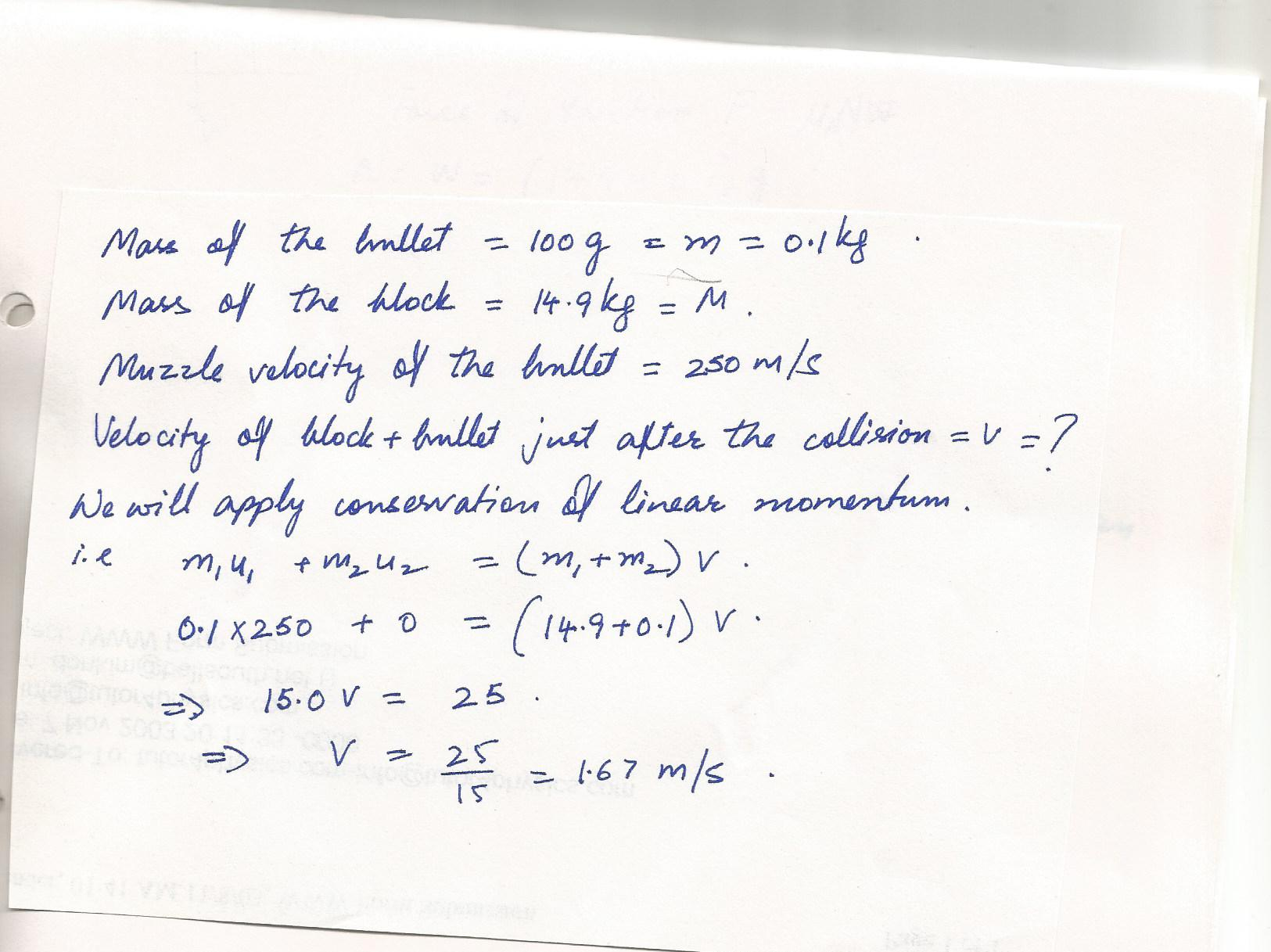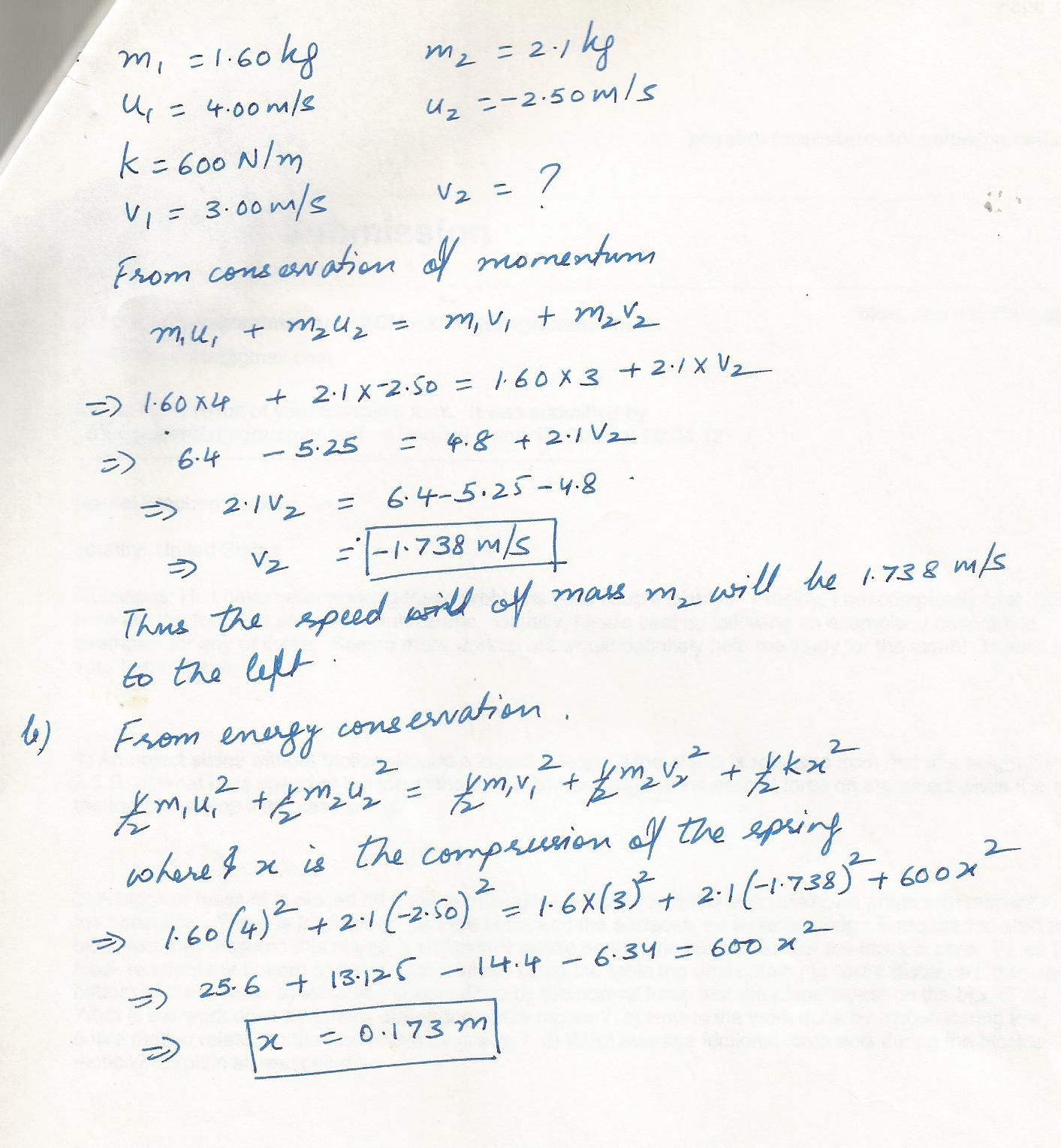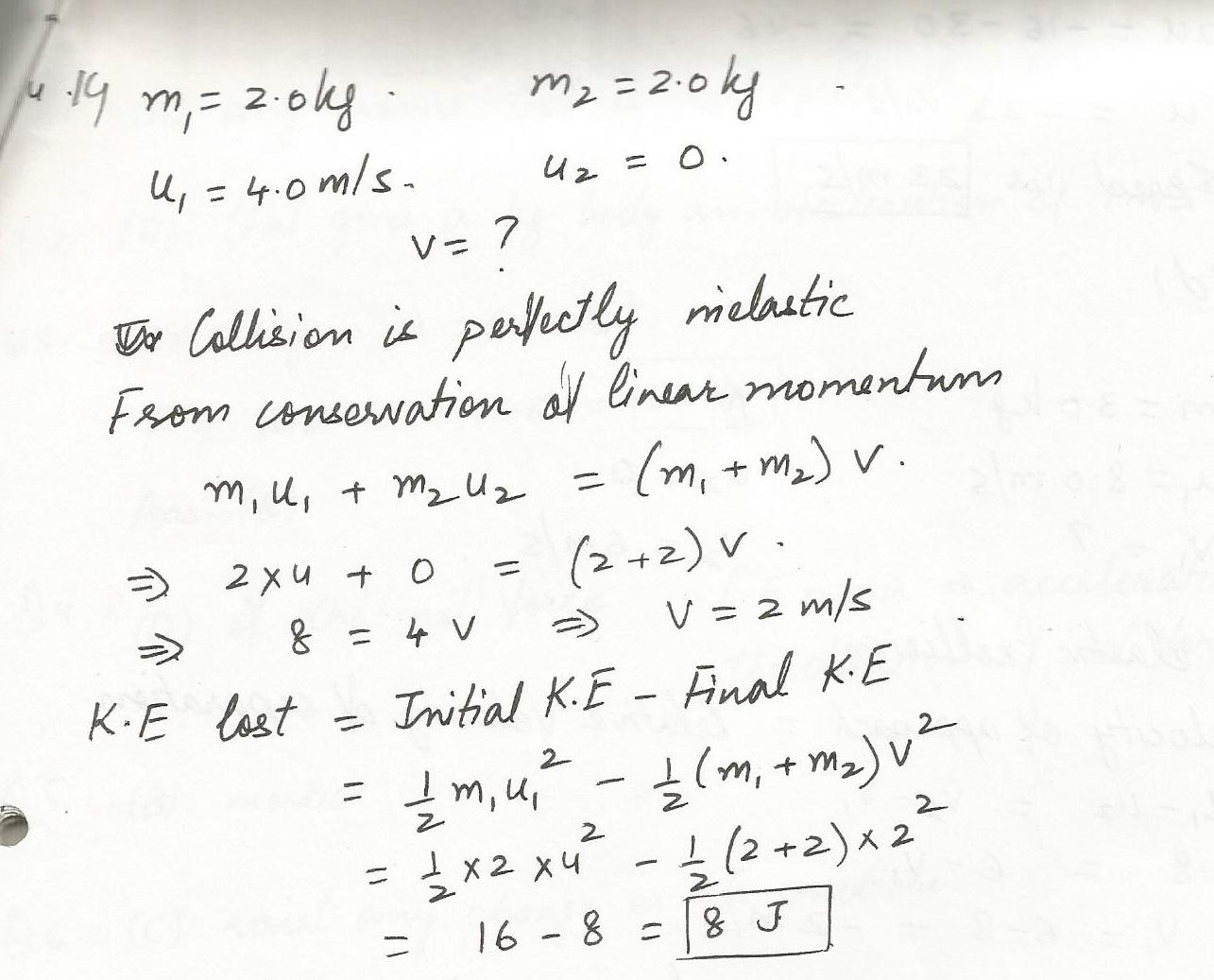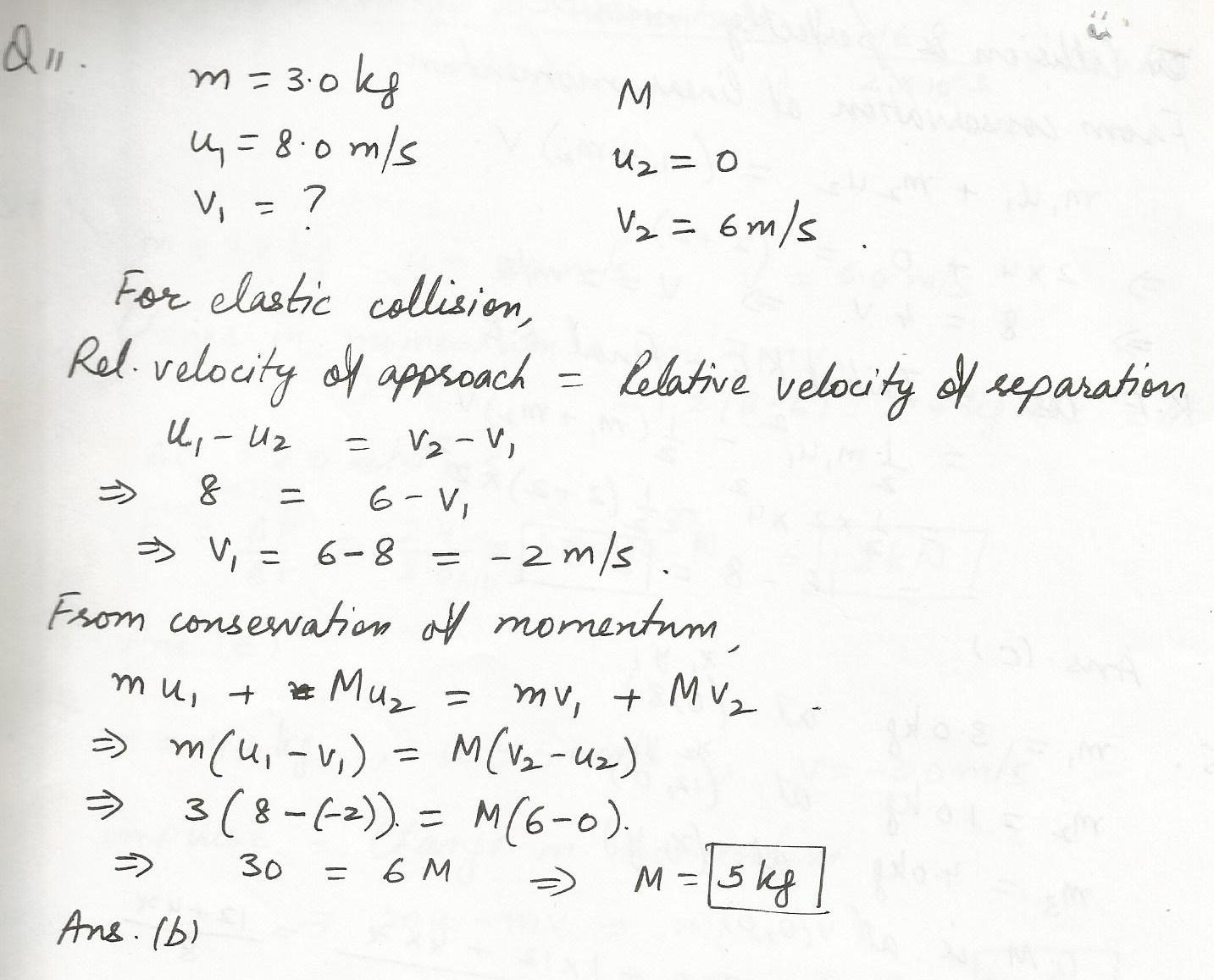8096

Physics Collisions Level: Misc Level

A bullet of mass 100 g is fired horizontally into a 14.9 kg block of wood resting on a horizontal surface, with the bullet becoming embedded in the block.If the muzzle velocity of the bullet is 250 m/s, what is the velocity of the block containing the bullet immediately after the collision? If the coefficient of friction between the block and the surface is 0.60, how far will the block slide before coming to rest?8093

Physics Collisions Level: Misc Level

A bullet of mass 100 g is fired horizontally into a 14.9 kg block of wood resting on a horizontal surface, with the bullet becoming embedded in the block.If the muzzle velocity of the bullet is 250 m/s, what is the velocity of the block containing the bullet immediately after the collision? If the coefficient of friction between the block and the surface is 0.60, how far will the block slide before coming to rest?7641

Physics Collisions Level: Misc Level

A block of mass m1=1.60 kg, initially moving to the right with a speed of 4.00 m/s on a frictionless horizontal surface, collides with a spring attached to a second block of mass m2=2.1 kg,moving to the left with a speed of 2.50 m/s. The spring has a spring constant of 600 N/m.a) At the instant when m1 is moving to the right with speed 3.00 m/s, determine the speed of m2. b) Determine the compression of the spring at the same moment listed in (a) above.7640

Physics Collisions Level: Misc Level

A block of mass m1=1.60 kg, initially moving to the right with a speed of 4.00 m/s on a frictionless horizontal surface, collides with a spring attached to a second block of mass m2=2.1 kg,moving to the left with a speed of 2.50 m/s. The spring has a spring constant of 600 N/m.a) At the instant when m1 is moving to the right with speed 3.00 m/s, determine the speed of m2. b) Determine the compression of the spring at the same moment listed in (a) above.6786

Physics Collisions Level: Misc Level

A 2.0-kg mass moving to the east at a speed of 4.0 m/s collides head-on in a perfectly inelastic collisision with a stationary 2.0-kg mass. How much kinetic energy is lost during this collision?

a.16J

b.4.0 J

c.8.0 J

d. zero6783

Physics Collisions Level: Misc Level

A 3.0-kg object moving 8.0 m/s in the positive x direction has a one- dimensional elastic collision with an object (mass=M)initially at rest.After the collision the object of unknown mass has a velocity of 6.0 m/s in the positive x direction. What is M?

a. 7.5 kg

b.5.0 kg

c.6.0 kg

d.4.2 kg

e.8.0 kg6782

Physics Collisions Level: Misc Level

A 6.0-kg object moving 5.0 m/s collides with and sticks to a 2.0-kg object.After the collsion the composite object is moving 2.0 m/s in a direction opposite to the initial direction of motion of the 6.0 -kg object. Determine the speed of the 2.0-kg object before the collision.

a.15 m/s

b.7.0 m/s

c.8.0 m/s

d.23 m/s

e.11 m/sDisplaying 1-7 of 7 results.